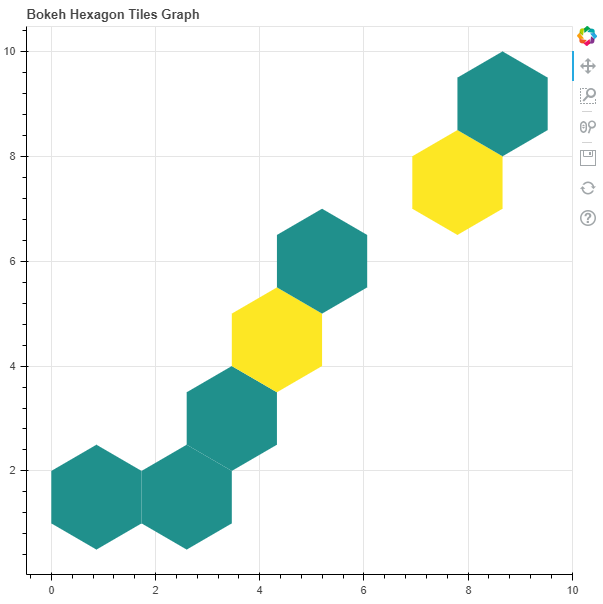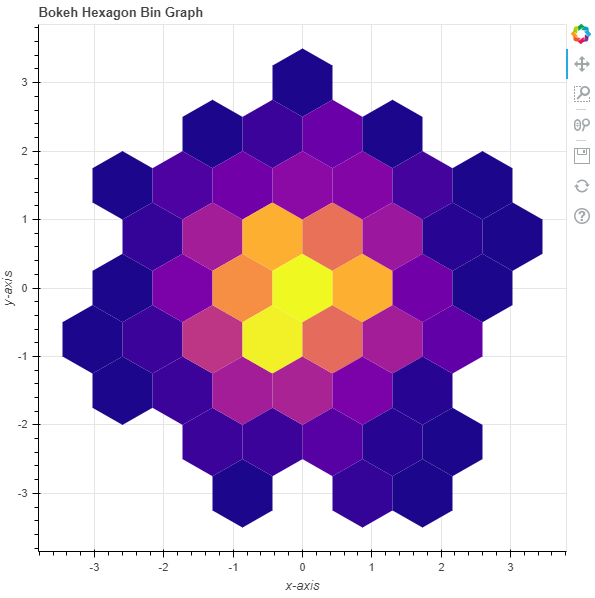# Python Bokeh – Plotting Hexagon Bins on a Graph

Bokeh is a Python interactive data visualization. It renders its plots using HTML and JavaScript. It targets modern web browsers for presentation providing elegant, concise construction of novel graphics with high-performance interactivity.

Bokeh can be used to plot hexagon tiles on a graph. Plotting hexagon tiles on a graph can be done using the `hexbin()` method of the `plotting` module.

## plotting.figure.hexbin()

Syntax : hexbin(parameters)

Parameters :

• x : NumPy array of x-coordinates of the hexagon tile bins
• y : NumPy array of y-coordinates of the hexagon tile bins
• size : radius of the hexagonal tile bins, default is 1
• palette : orientation value, default is Viridis256
• orientation : palette to colormap the bins, default is pointytop
• line_color : color of the line, default is black
• fill_color : fill color value of the hexagon tile bin markers
• aspect_scale : aspect ratio scaling value

Any additional keyword arguments are passed to `hex_tile()`.

Returns : an object of class `GlyphRenderer` and `DataFrame`

Example 1 : In this example we will be using the default values for plotting the graph.

 `# importing the modules  ` `from` `bokeh.plotting ``import` `figure, output_file, show  ` `import` `numpy as np ` ` `  `# file to save the model  ` `output_file(``"gfg.html"``)  ` `      `  `# instantiating the figure object  ` `graph ``=` `figure(title ``=` `"Bokeh Hexagon Bin Graph"``)  ` `   `  `# the points to be plotted  ` `x ``=` `np.array([``1``, ``2``, ``3``, ``4``, ``5``, ``6``, ``7``, ``8``, ``9``]) ` `y ``=` `np.array([``1``, ``2``, ``3``, ``4``, ``5``, ``6``, ``7``, ``8``, ``9``]) ` ` `  `# plotting the graph  ` `graph.hexbin(x, y, size ``=` `1``)  ` `    `  `# displaying the model  ` `show(graph)  `

Output :Example 2 : In this example we will be plotting the hexagon bins with various parameters

 `# importing the modules  ` `from` `bokeh.plotting ``import` `figure, output_file, show  ` `import` `numpy as np ` ` `  `# file to save the model  ` `output_file(``"gfg.html"``)  ` `      `  `# instantiating the figure object  ` `graph ``=` `figure(title ``=` `"Bokeh Hexagon Bin Graph"``)  ` ` `  `# name of the x-axis  ` `graph.xaxis.axis_label ``=` `"x-axis"` `      `  `# name of the y-axis  ` `graph.yaxis.axis_label ``=` `"y-axis"` `   `  `# the points to be plotted  ` `x ``=` `np.random.standard_normal(``500``) ` `y ``=` `np.random.standard_normal(``500``) ` ` `  `# size of the bins ` `size ``=` `0.5` ` `  `# color palette of the graph ` `palette ``=` `"Plasma256"` ` `  `# plotting the graph  ` `graph.hexbin(x, y, ` `             ``size ``=` `0.5``, ` `             ``palette ``=` `palette)  ` `    `  `# displaying the model  ` `show(graph)   `

Output :My Personal Notes arrow_drop_upCheck out this Author's contributed articles.

If you like GeeksforGeeks and would like to contribute, you can also write an article using contribute.geeksforgeeks.org or mail your article to contribute@geeksforgeeks.org. See your article appearing on the GeeksforGeeks main page and help other Geeks.

Please Improve this article if you find anything incorrect by clicking on the "Improve Article" button below.

Article Tags :

Be the First to upvote.

Please write to us at contribute@geeksforgeeks.org to report any issue with the above content.